Next: Transverse Standing Waves Up: Coupled Oscillations Previous: Three Spring-Coupled Masses

# Exercises

1. A particle of mass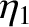is attached to a rigid support by means of a spring of spring constant. At equilibrium, the spring hangs vertically downward. An identical oscillator is added to this system, the spring of the former being attached to the mass of the latter. Calculate the normal frequencies for one-dimensional vertical oscillations about the equilibrium state, and describe the associated normal modes.

2. Consider a mass-spring system of the general form shown in Figure 15 in which the two masses are of mass, the two outer springs have spring constant, and the middle spring has spring constant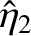. Find the normal frequencies and normal modes in terms of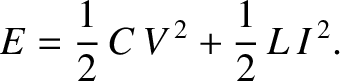and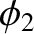.

3. Consider a mass-spring system of the general form shown in Figure 15 in which the two masses are of mass, the two leftmost springs have spring constant, and the rightmost spring is absent. Find the normal frequencies and normal modes in terms of.

4. Consider a mass-spring system of the general form shown in Figure 15 in which the springs all have spring constant, and the left and right masses are of massand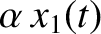, respectively. Find the normal frequencies and normal modes in terms ofand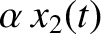.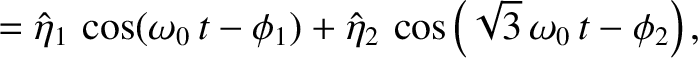5. Find the normal frequencies and normal modes of the coupled LC circuit shown in the preceding figure terms of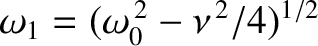and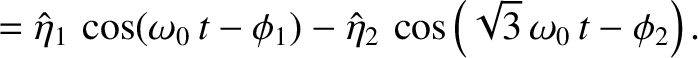.

6. Consider two simple pendula with the same length,, but different bob masses,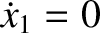and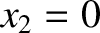. Suppose that the pendula are connected by a spring of spring constant. Let the spring be unextended when the two bobs are in their equilibrium positions. Demonstrate that the equations of motion of the system (for small amplitude oscillations) are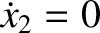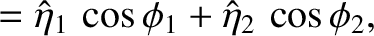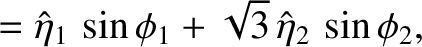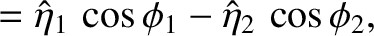where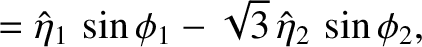and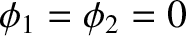are the angular displacements of the respective pendula from their equilibrium positions. Show that the normal coordinates are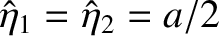and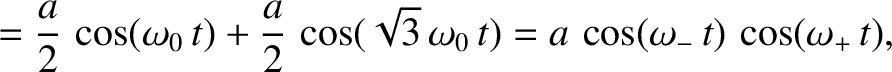. Find the normal frequencies and normal modes. Find a superposition of the two modes such that atthe two pendula are stationary, with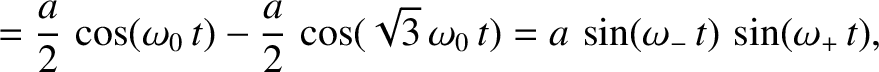, and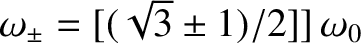.

7. A linear triatomic molecule (e.g., carbon dioxide) consists of a central atom of mass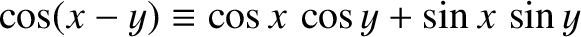flanked by two identical atoms of mass. The atomic bonds are represented as springs of spring constant. Find the molecule's normal frequencies and modes of linear oscillation.Next: Transverse Standing Waves Up: Coupled Oscillations Previous: Three Spring-Coupled Masses
Richard Fitzpatrick 2013-04-08